# poseplot

## Syntax

``poseplot``
``poseplot(quat)``
``poseplot(R)``
``poseplot(___,position)``
``poseplot(___,frame)``
``poseplot(___,Name=Value)``
``poseplot(ax,___)``
``p = poseplot(___)``

## Description

example

````poseplot` plots the pose (position and orientation) at the coordinate origin position with zero rotation. The default navigation frame is the north-east-down (NED) frame.```

example

````poseplot(quat)` plots the pose with orientation specified by a quaternion `quat`. The position by default is ```[0 0 0]```. ```

example

````poseplot(R)` plots the pose with orientation specified by a rotation matrix `R`. The position by default is ```[0 0 0]```. ```

example

````poseplot(___,position)` specifies the position of the pose plot. ```
````poseplot(___,frame)` specifies the navigation frame of the pose plot. ```

example

````poseplot(___,Name=Value)` specifies pose patch properties using one or more name-value arguments. For example, `poseplot(PatchFaceColor="r")` plots the pose with red face color. For a list of properties, see PosePatch Properties. ```
````poseplot(ax,___)` specifies the parent axes of the pose plot.```

example

````p = poseplot(___)` returns the `PosePatch` object. Use `p` to modify properties of the pose patch after creation. For a list of properties, see PosePatch Properties.```

## Examples

collapse all

Plot the default pose using the `poseplot` function with default settings.

```poseplot xlabel("North-x (m)") ylabel("East-y (m)") zlabel("Down-z (m)");```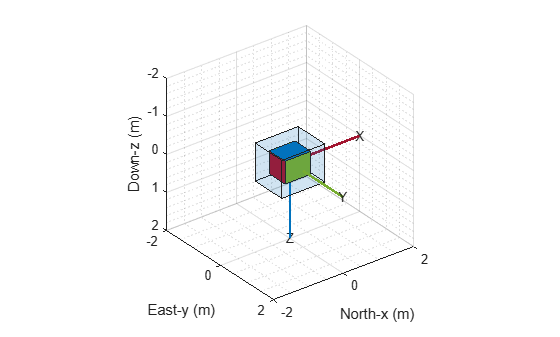Next, plot a pose with specified orientation and position.

```q = quaternion([35 10 50],"eulerd","ZYX","frame"); position = [1 1 1]; poseplot(q,position)```Then, plot a second pose on the figure and return the `PosePatch` object. Plot the second pose with a smaller size by using the `ScaleFactor` name-value argument.

```hold on p = poseplot(eye(3),[5 5 5],ScaleFactor=0.5)```
```p = PosePatch with properties: Orientation: [3x3 double] Position: [5 5 5] Show all properties ```
```legend("First Pose","Second Pose") hold off```Animate a series of poses using the `poseplot` function. First, define the initial and final positions.

```ps = [0 0 0]; pf = [10 0 0];```

Then, define the initial and final orientations using the `quaternion` object.

```qs = quaternion([45,0,0],'eulerd','ZYX','frame'); qf = quaternion([-45,0,0],'eulerd','ZYX','frame');```

Show the starting pose.

```patch = poseplot(qs,ps); ylim([-2 2]) xlim([-2 12]) xlabel("North-x (m)") ylabel("East-y (m)") zlabel("Down-z (m)");```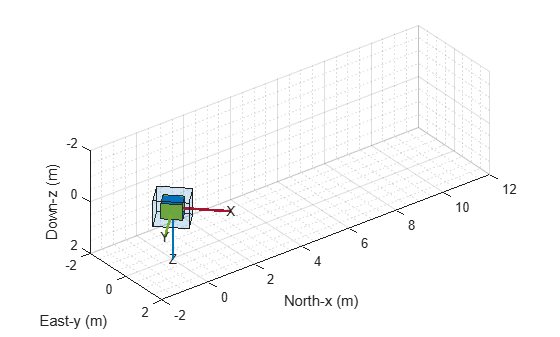Change the position and orientation continuously using coefficients, and update the pose using the `set` object function.

```for coeff = 0:0.01:1 q = slerp(qs,qf,coeff); position = ps + (pf - ps)*coeff; set(patch,Orientation=q,Position=position); drawnow end```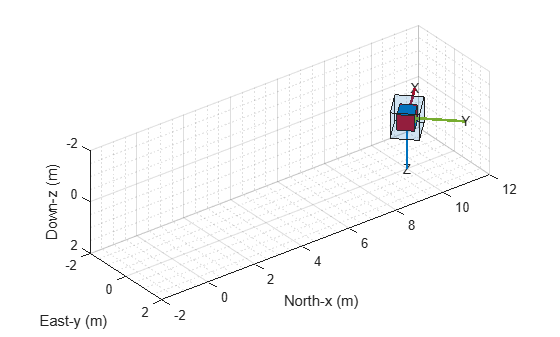Plot orientations and positions in meshes using the `poseplot` function. First, plot a ground vehicle at the origin with zero rotation.

```poseplot(ones("quaternion"),[0 0 0],MeshFileName="groundvehicle.stl",ScaleFactor=0.3); xlabel("North-x (m)") ylabel("East-y (m)") zlabel("Down-z (m)")```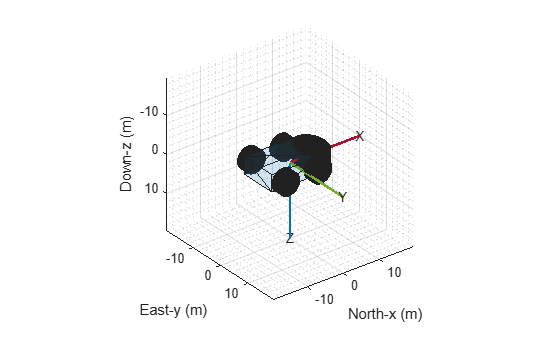Second, plot a rotor at the position `[20 20 -20]` with zero rotation.

```hold on poseplot(ones("quaternion"),[20 20 -20],MeshFileName="multirotor.stl",ScaleFactor=0.2);```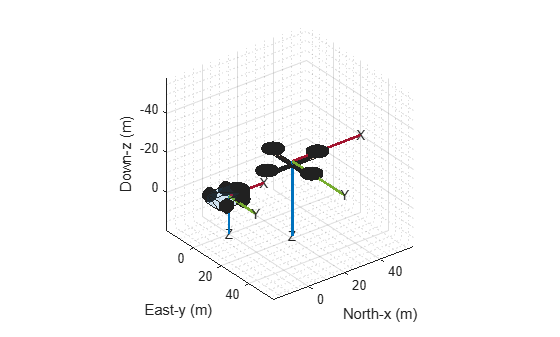Lastly, plot a fixed-wing aircraft at the position `[5 5 -40] `with zero rotation.

```poseplot(ones("quaternion"),[5 5 -40],MeshFileName="fixedwing.stl",ScaleFactor=0.4); view([-37.8 28.4]) hold off```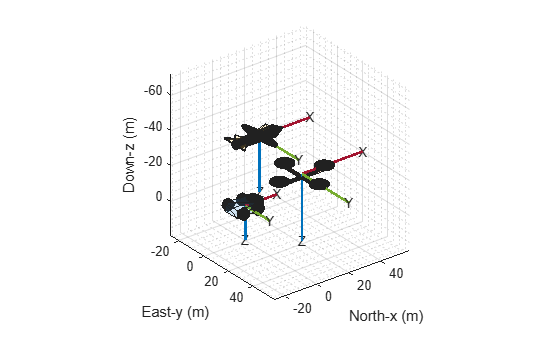## Input Arguments

collapse all

Quaternion, specified as a `quaternion` object.

Rotation matrix, specified as a 3-by-3 orthonormal matrix.

Example: `eye(3)`

Position of the pose plot, specified as a three-element real-valued vector.

Example: `[1 3 4]`

Navigation frame of the pose plot, specified as `"NED"` for the north-east-down frame or `"ENU"` for the east-north-up frame.

When the parent axes status is `hold off`, specifying the NED navigation frame reverses the y- and z-axes in the figure by setting the `YDir` and `ZDir` properties of the parent axes.

Parent axes of the pose plot, specified as an `Axes` object. If you do not specify the axes, the `poseplot` function uses the current axes.

### Name-Value Arguments

Specify optional pairs of arguments as `Name1=Value1,...,NameN=ValueN`, where `Name` is the argument name and `Value` is the corresponding value. Name-value arguments must appear after other arguments, but the order of the pairs does not matter.

Example: `poseplot(PatchFaceAlpha=0.1)`

The `PosePatch` properties listed here are only a subset. For a complete list, see PosePatch Properties.

Orientation of the pose plot, specified as a `quaternion` object or a rotation matrix.

Position of the pose plot, specified as a three-element real-valued vector.

Name of Standard Triangle Language (STL) mesh file, specified as a string scalar or a character vector containing the name of the mesh file. When you specify this argument, the `poseplot` function plots the mesh instead of the orientation box.

Scale factor of the pose plot, specified as a nonnegative scalar. The scale factor controls the size of the orientation box. When you specify the `MeshFileName` argument, the scale factor also changes the scale of the mesh.

Patch face color, specified as an RGB triplet, a hexadecimal color code, a color name, or a short name.

• An RGB triplet is a three-element row vector whose elements specify the intensities of the red, green, and blue components of the color. The intensities must be in the range `[0, 1]`; for example, `[0.4 0.6 0.7]`.

• A hexadecimal color code is a character vector or a string scalar that starts with a hash symbol (`#`) followed by three or six hexadecimal digits, which can range from `0` to `F`. The values are not case sensitive. Thus, the color codes `"#FF8800"`, `"#ff8800"`, `"#F80"`, and `"#f80"` are equivalent.

Here is a list of commonly used colors and their corresponding values.

Color NameShort NameRGB TripletHexadecimal Color CodeAppearance
`"red"``"r"``[1 0 0]``"#FF0000"``"green"``"g"``[0 1 0]``"#00FF00"``"blue"``"b"``[0 0 1]``"#0000FF"``"cyan"` `"c"``[0 1 1]``"#00FFFF"``"magenta"``"m"``[1 0 1]``"#FF00FF"``"yellow"``"y"``[1 1 0]``"#FFFF00"``"black"``"k"``[0 0 0]``"#000000"``"white"``"w"``[1 1 1]``"#FFFFFF"`Patch face transparency, specified as a scalar in range `[0, 1]`. A value of `1` is fully opaque and `0` is completely transparent.

## Output Arguments

collapse all

Pose patch object, returned as a `PosePatch` object. You can use the returned object to query and modify properties of the plotted pose. For a list of properties, see PosePatch Properties.